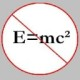You are currently browsing the tag archive for the ‘cesaro’ tag.

The following theorem, I feel, is not very well-known, though it is a particularly useful one for solving certain types of “limit” problems. Let me pose a couple of elementary problems and offer their solutions. First, the theorem.

Stolz-Cesàro: Let$(a_n)_{n \ge 1}$ and$(b_n)_{n \ge 1}$ be two sequences of real numbers, such that$(b_n)$ is positive, strictly increasing and unbounded. Then,$\displaystyle \lim_{n \to \infty} \frac{a_n}{b_n} = \lim_{n \to \infty} \frac{a_{n+1} - a_n}{b_{n+1} - b_n}$,

if the limit on the right hand side exists.

The proof involves the usual$\epsilon - \delta$ method, and I will avoid presenting it here since it isn’t particularly interesting. Just as Abel’s lemma is the discrete analogue of integration by parts, the Stolz-Cesàro theorem may be considered the discrete analogue of L’Hospital’s rule in calculus.

Problem 1: Evaluate the limit$\displaystyle \lim_{n \to \infty} \frac{1^k + 2^k + \ldots + n^k}{n^{k+1}}$, where$k \in \mathbb{N}$.

Solution: One may certainly consider the above limit as a Riemann-sum which may then be transformed into the integral$\displaystyle \int_0^1 x^k \, dx$, which then obviously evaluates to$1/(k+1)$. But, we will take a different route here.

First, let$a_n = 1^k + 2^k + \ldots + n^k$ and$b_n = n^{k+1}$. Then, we note that the sequence$(b_n)$ is positive, strictly increasing and unbounded. Now,$\displaystyle \lim_{n \to \infty} \frac{a_{n+1} - a_n}{b_{n+1} - b_n} = \lim_{n \to \infty} \frac{(n+1)^k}{(n+1)^{k+1} - n^{k+1}}$$\displaystyle = \lim_{n \to \infty} \frac{(n+1)^k}{\left(1 + \binom{k+1}{1}n + \binom{k+1}{2}n^2 + \ldots + \binom{k+1}{k}n^k + n^{k+1}\right) - n^{k+1}}$

(using the binomial theorem)$\displaystyle = \lim_{n \to \infty} \frac{(n+1)^k / n^k}{\left(1 + \binom{k+1}{1}n + \binom{k+1}{2}n^2 + \ldots + \binom{k+1}{k}n^k \right) / n^k}$$\displaystyle = \lim_{n \to \infty} \frac{(1 + 1/n)^k}{\binom{k+1}{k}} = \frac1{k+1}$.

Therefore, using the Stolz-Cesàro theorem, we conclude that the required limit is also$1/(k+1)$.

Let us now look at another problem where applying the aforesaid theorem makes our job a lot easier. This problem is an example of one that is not amenable to the other usual methods of evaluating limits.

Problem 2: Let$k\geq 2$ be integers and suppose$C: y = \sqrt {2x + 1}\ (x > 0)$. Given the tangent line at the point$(a_{k},\, \sqrt {2a_{k} + 1})$ from the point$(0, k)$ to$C$, evaluate$\displaystyle \lim_{n\to\infty}\frac {1}{n^{3}}\sum_{k = 2}^{n}a_{k}$.

Solution:(This is basically the solution I had offered elsewhere a while ago; so, it’s pretty much copy/paste!)$\displaystyle y = \sqrt {2x + 1}\Rightarrow \frac {dy}{dx} = \frac {1}{\sqrt {2x + 1}}$.

So, the equation of the tangent line at the point$(a_{k}, \sqrt {2a_{k} + 1})$ is given by$\displaystyle y - \sqrt {2a_{k} + 1} = \frac {1}{\sqrt {2a_{k} + 1}}(x - a_{k}).$

Since the point$(0,k)$ lies on this line, we must have$\displaystyle k - \sqrt {2a_{k} + 1} = \frac {1}{\sqrt {2a_{k} + 1}}( - a_{k})$

The above, after squaring and some algebraic manipulation yields$a_{k}^{2} + 2(1 - k^{2})a_{k} + 1 - k^{2} = 0$, which implies$a_{k} = (k^{2} - 1) + k(\sqrt {k^{2} - 1})$. We drop the negative root because$a_{k} > 0$ for all$k\ge 2$.

(This is where the Stolz-Cesàro theorem actually comes into play!)

Now, let$(b_{n})$ and$(c_{n})$ be two sequences such that$\displaystyle b_{n} = \sum_{k = 2}^{n}a_{k}$ and$c_{n} = n^{3}.$
Note that$(c_{n})$ is a positive, increasing and unbounded sequence.

Therefore,$\displaystyle \lim_{n\to \infty}\frac {b_{n + 1} - b_{n}}{c_{n + 1} - c_{n}} = \lim_{n\rightarrow \infty}\frac {\sum_{k = 2}^{n + 1}a_{k} - \sum_{k = 2}^{n}a_{k}}{(n + 1)^{3} - n^{3}} = \lim_{n\rightarrow \infty}\frac {a_{n + 1}}{3n^{2} + 3n + 1}$$\displaystyle = \lim_{n\to \infty}\frac {(n + 1)^{2} - 1 + (n + 1)\sqrt {(n + 1)^{2} - 1}}{3n^{2} + 3n + 1}$$\displaystyle = \lim_{n \to \infty} \frac{(1 + 1/n)^2 - 1/n^2 + (1 + 1/n)\sqrt{1 + 2/n}}{3 + 3/n + 1/n^2}$$= 2/3$.

Therefore, by the Stolz- Cesàro theorem, we have$\displaystyle \lim_{n\to \infty}\frac {b_{n}}{c_{n}} = 2/3 \,$, and so$\displaystyle \lim_{n\to \infty}\frac {1}{n^{3}}\sum_{k = 2}^{n}a_{k} = 2/3$.

### Blog Stats

• 346,162 hitsprof dr drd horia or… on My first postprof drd horia orasa… on My first postprof dr mircea orasa… on Inequality with lognotedscholar on Self-referential Paradoxes, In…prof dr mircea orasa… on Inequality with logprof dr mircea orasa… on Inequality with logprof dr mircea orasa… on 2010 in reviewkenji on Basic category theory, Iprof dr mircea orasa… on Solution to POW-10: Another ha…prof drd horia orasa… on Continued fraction for eprof drd horia orasa… on Inequality with logprof dr mircea orasa… on Solution to POW-12: A graph co…prof dr mircea orasa… on The Character of Physical…prof dr mircea orasa… on Milk and Tea puzzleprof drd horia orasa… on Inequality with log